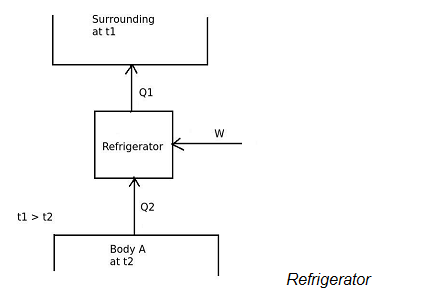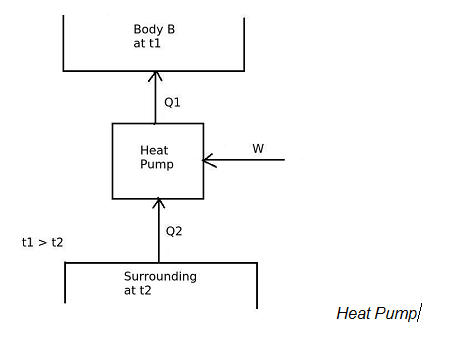# Second Law of Thermodynamics - Mechanical Engineering (MCQ) questions and answers

1)   Which among the following is correct relation between COP of heat pump and COP of refrigerator?

a. [COP]H.P. = 1 + [COP]ref
b. [COP]H.P. = 1 – [COP]ref
c. [COP]H.P. = [COP]ref
d. none of the above
 Answer  Explanation ANSWER: [COP]H.P. = 1 + [COP]ref Explanation: The COP of a device, operating in a cycle is given by,[COP] = Desired effect / Work inputIn refrigerator,the desired effect is to maintain temperature of the body A lower than the surrounding temperature.Therefore, A refrigerator is a device, which operates in a cycle and maintains the temperature a particular body lower than the surrounding temperature.[COP]ref = Desired effect / Work input[COP]ref = Q2 / W[COP]H.PAnd in heat pump,the desired effect is to maintain temperature of the body B more than the surrounding temperature.Therefore, [COP]H.P. = Desired effect / Work input[COP]H.P = Q1 / Wand the work input is given by, W = Q1 – Q2 From the equations of [COP]ref and [COP]H.P ,[COP]H.P. = 1 + [COP]ref

2)   The irreversibility of a process occurs due to

a. lack of equilibrium during the process
b. involvement of dissipative effects
c. either a. or b. or both
d. none of the above
 Answer  Explanation ANSWER: either a. or b. or both Explanation: A reversible process is carried out infinitely slowly, so that every state passed through by the system is an equilibrium state. In the irreversible process every state will not be an equilibrium state as it is carried out very fast. Only the first and the last state of the irreversible process are the equilibrium states. Therefore lack of equilibrium is a cause of irreversibility. In a dissipative effect the energy is transformed from one form to another form and the final form of energy has a less capacity to do mechanical work. Thus this process becomes irreversible. Therefore involvement of dissipative effect is another cause of irreversibility.

3)   Efficiency of heat engine cycle is the ratio of

a. total heat input to the cycle (Qin) to net work output of the cycle (Wnet)
b. net work output of the cycle (Wnet) to total heat input to the cycle (Qin)
c. net work output of the cycle (Wnet) to heat rejected from the system (Qout)
d. none of the above
 Answer  Explanation ANSWER: total heat input to the cycle (Qin) to net work output of the cycle (Wnet) Explanation: In heat engine cycle, the heat is transferred to the system and work is transferred from the system. The main function of heat engine is to produce work output continuously by consuming heat energy given to the system. So the net work (Wnet) and heat supplied (Qin) to the system are taken into account. The net work output is the difference between work produces in the process and the work input required to take the system to initial state. In stem power plant, net work output is,Turbine Work (WT) – Pump Work (WP)Therefore, the efficiency of heat engine cycle is the ratio of total heat input to the cycle (Qin) to net work output of the cycle (Wnet).

4)   PMM2 is the machine which violates ___________

a. Kelvin-Planck statement
b. Clausius statement
c. both a. and b.
d. none of the above
 Answer  Explanation ANSWER: both a. and b. Explanation: PMM2 (Perpetual Motion Machine of the Second Kind) is a hypothetical machine which violates the second law of thermodynamics. Kelvin-Planck as well as Clausius statement both represents the second law of thermodynamics. The Kelvin-Planck statement is related to heat engine whereas the Clausius statement is related to heat pump.If a machine violates one of the two statements then the other statement is always violated. Therefore, PMM2 is the machine which violates both Kelvin-Planck as well as Clausius statement of second law of thermodynamics.

5)   A cyclic heat engine operates between a source temperature of 927 oC and a sink temperature of 27 oC. What will be the maximum efficiency of the heat engine?

a. 100 %
b. 80 %
c. 75 %
d. 70 %
 Answer  Explanation ANSWER: 75 % Explanation: Heat engine is operated between the temperaturesT1 = 927 0C = 927 + 273 = 1200 K andT2 = 27 0C = 27 + 273 = 300 KThe maximum efficiency of a heat engine is given by,ηmax = 1 – (T2/T1)ηmax = 1 – (300/1200)ηmax = 0.75

6)   Match the following irreversibilities

(1) Mechanical irreversibility ------ (A) Refrigeration cycle
(2) Thermal irreversibility --------- (B) Forming of water by combination of Hydrogen and Oxygen
(3) Chemical irreversibility -------- (C) Free expansion of gas in a system
(4) External irreversibility --------- (D) Melting of ice cube under the sunlight

a. (1)-(A), (2)-(B), (3)-(C), (4)-(D)
b. (1)-(A), (2)-(D), (3)-(B), (4)-(C)
c. (1)-(C), (2)-(D), (3)-(A), (4)-(B)
d. (1)-(C), (2)-(D), (3)-(B), (4)-(A)
 Answer  Explanation ANSWER: (1)-(C), (2)-(D), (3)-(B), (4)-(A) Explanation: If the irreversibility is due to a finite pressure gradient, it is called as mechanical irreversibility. Thus, free expansion of gas in a system is the example of mechanical irreversibility. It the irreversibility is due to a finite temperature gradient, it is called as thermal irreversibility. Thus, melting of ice cube under the sunlight is the example of mechanical irreversibility. If the irreversibility is due to a finite concentration gradient or chemical reaction in the system, it is called as chemical irreversibility. Thus, forming of water by combination of Hydrogen and Oxygen is the example of mechanical irreversibility.In refrigeration cycle, irreversibility occurs due to temperature difference between the source and a working fluid that is refrigerant at heat supply and the temperature difference between the sink and the refrigerant at heat rejection.

7)   When a process becomes irreversible due to heat interaction between system and surrounding at the boundary due to finite temperature gradient, then the irreversibility is______

a. internal irreversibility
b. external irreversibility
c. mechanical irreversibility
d. chemical irreversibility
 Answer  Explanation ANSWER: external irreversibility Explanation: All the spontaneous processes are irreversible in nature. The irreversibility is caused by finite potential gradient like temperature gradient etc. or by any dissipative effect like friction. There are two types of irreversibilityi.) Internal irreversibilityii.) External irreversibilityThe external irreversibility occurs due to the temperature difference between the source and a working fluid at heat supply and the temperature difference between the sink and the working fluid at heat rejection. If the hypothetical heat source and sink is considered then the process becomes reversible.

8)   The irreversibility in the system caused by friction is an example of

a. internal irreversibility
b. external irreversibility
c. frictional irreversibility
d. chemical irreversibility
 Answer  Explanation ANSWER: internal irreversibility Explanation: All the spontaneous processes are irreversible in nature. The irreversibility is caused by finite potential gradient like temperature gradient etc. or by any dissipative effect like friction. There are two types of irreversibilityi. Internal irreversibilityii. External irreversibilityInternal irreversibility is caused by internal dissipative effects in the system like friction, turbulence, electric resistance etc. Thus the irreversibility in the system caused by friction is an example of internal irreversibility.

9)   Assume that a reversible heat engine is operating between a source at T1 and a sink at T2. If T2 decreases, the efficiency of the heat engine  ______

a. decreases
b. increases
c. remains constant
d. none of the above
 Answer  Explanation ANSWER: increases Explanation: The efficiency of a reversible heat engine is operating between a source at T1 and a sink at T2 is given by,η = Work done / Heat suppliedηrev = ηmax = ( T1 – T2 ) / T1ηrev = 1 – (T2/T1)From the above equation, as T2 decreases the efficiency increases and tends towards 100%

10)   Any attainable value of absolute temperature is ________

a. always less than zero
b. always equals to zero
c. always greater than zero
d. none of the above
 Answer  Explanation ANSWER: always greater than zero Explanation: It is impossible for a system to undergo a reversible isothermal process without transfer of heat. To make this process possible, the temperature required is an absolute zero temperature and the second law of thermodynamics is also violated. Thus any attainable value of absolute temperature is always greater than zero. This is also called as third law of thermodynamics. It states that it is impossible for any process to reduce any system to an absolute zero temperature in a finite number of operations.

1 2 3 4 5